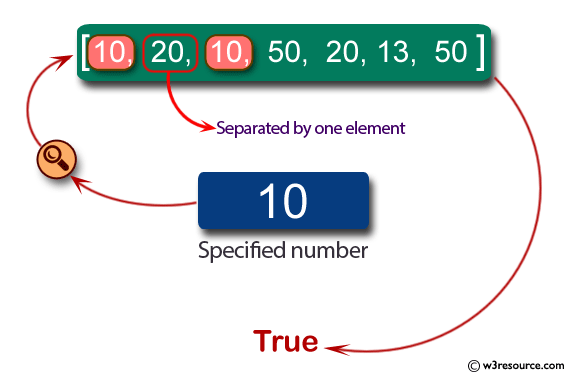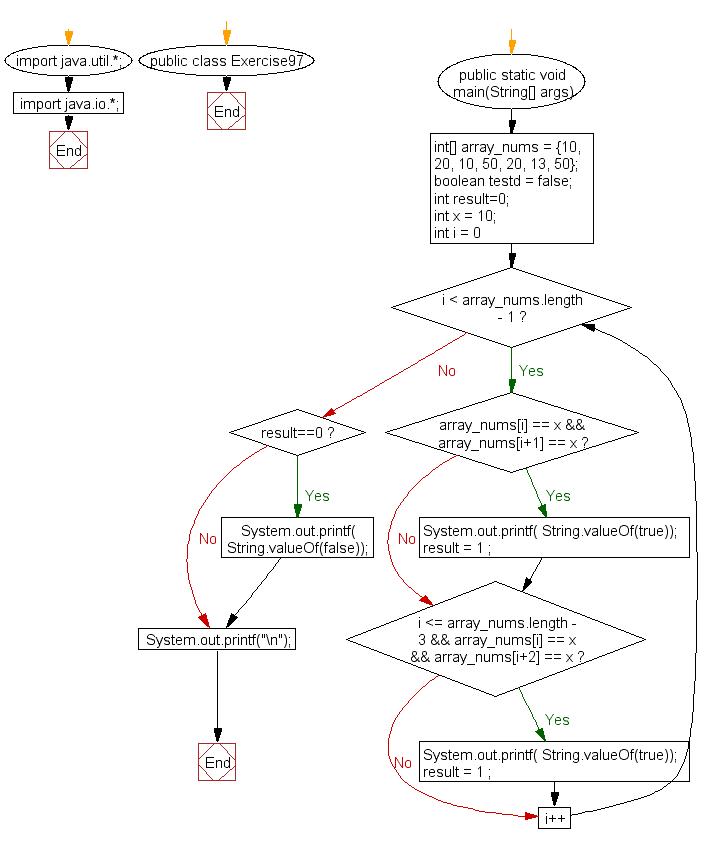﻿ Java: Check if an array contains a value next to each other# Java Exercises: Check if an array of integers contains a specified number next to each other or there are two same specified numbers separated by one element

## Java Basic: Exercise-97 with Solution

Write a Java program to check if an array of integers contains a specified number next to each other or there are two same specified numbers separated by one element.

Pictorial Presentation:Sample Solution:

Java Code:

``````import java.util.*;
import java.io.*;
public class Exercise97 {
public static void main(String[] args)
{
int[] array_nums = {10, 20, 10, 50, 20, 13, 50};
//int[] array_nums = {10, 10, 50, 50, 20, 13, 50};
boolean testd = false;
int result=0;
int x = 10;

for(int i = 0; i < array_nums.length - 1; i++) {
if(array_nums[i] == x && array_nums[i+1] == x)
{
System.out.printf( String.valueOf(true));
result = 1 ;
}

if(i <= array_nums.length - 3 && array_nums[i] == x && array_nums[i+2] == x)
{
System.out.printf( String.valueOf(true));
result = 1 ;
}
}

if (result==0)
{
System.out.printf( String.valueOf(false));
}
System.out.printf("\n");
}
}
```
```

Sample Output:

```true
```

Flowchart:Java Code Editor:

What is the difficulty level of this exercise?

Test your Programming skills with w3resource's quiz.

﻿

## Java: Tips of the Day

countOccurrences

Counts the occurrences of a value in an array.

Use Arrays.stream().filter().count() to count total number of values that equals the specified value.

```public static long countOccurrences(int[] numbers, int value) {
return Arrays.stream(numbers)
.filter(number -> number == value)
.count();
}
```

Ref: https://bit.ly/3kCAgLb